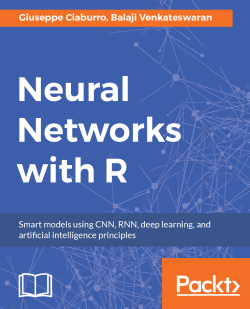# Neural Networks with R4.6 (7 reviews total)
By Giuseppe Ciaburro , Balaji Venkateswaran
• \$33.99 eBook
• \$41.99 Print + eBook
• \$12.99 eBook + Subscription
What do you get with a Packt Subscription?

• Constantly updated with 100+ new titles each month
• Breadth and depth in over 1,000+ technologies

Neural networks are one of the most fascinating machine learning models for solving complex computational problems efficiently. Neural networks are used to solve wide range of problems in different areas of AI and machine learning.

This book explains the niche aspects of neural networking and provides you with foundation to get started with advanced topics. The book begins with neural network design using the neural net package, then you’ll build a solid foundation knowledge of how a neural network learns from data, and the principles behind it. This book covers various types of neural network including recurrent neural networks and convoluted neural networks. You will not only learn how to train neural networks, but will also explore generalization of these networks. Later we will delve into combining different neural network models and work with the real-world use cases.

By the end of this book, you will learn to implement neural network models in your applications with the help of practical examples in the book.

Publication date:
September 2017
Publisher
Packt
Pages
270
ISBN
9781788397872

## Chapter 1. Neural Network and Artificial Intelligence Concepts

From the scientific and philosophical studies conducted over the centuries, special mechanisms have been identified that are the basis of human intelligence. Taking inspiration from their operations, it was possible to create machines that imitate part of these mechanisms. The problem is that they have not yet succeeded in imitating and integrating all of them, so the Artificial Intelligence (AI) systems we have are largely incomplete.

A decisive step in the improvement of such machines came from the use of so-called Artificial Neural Networks (ANNs) that, starting from the mechanisms regulating natural neural networks, plan to simulate human thinking. Software can now imitate the mechanisms needed to win a chess match or to translate text into a different language in accordance with its grammatical rules.

This chapter introduces the basic theoretical concepts of ANN and AI. Fundamental understanding of the following is expected:

• Basic high school mathematics; differential calculus and functions such as sigmoid
• R programming and usage of R libraries

We will go through the basics of neural networks and try out one model using R. This chapter is a foundation for neural networks and all the subsequent chapters.

We will cover the following topics in this chapter:

• ANN concepts
• Neurons, perceptron, and multilayered neural networks
• Bias, weights, activation functions, and hidden layers
• Forward and backpropagation methods
• Brief overview of Graphics Processing Unit (GPU)

At the end of the chapter, you will be able to recognize the different neural network algorithms and tools which R provides to handle them.

## Introduction

The brain is the most important organ of the human body. It is the central processing unit for all the functions performed by us. Weighing only 1.5 kilos, it has around 86 billion neurons. A neuron is defined as a cell transmitting nerve impulses or electrochemical signals. The brain is a complex network of neurons which process information through a system of several interconnected neurons. It has always been challenging to understand the brain functions; however, due to advancements in computing technologies, we can now program neural networks artificially.

The discipline of ANN arose from the thought of mimicking the functioning of the same human brain that was trying to solve the problem. The drawbacks of conventional approaches and their successive applications have been overcome within well-defined technical environments.

AI or machine intelligence is a field of study that aims to give cognitive powers to computers to program them to learn and solve problems. Its objective is to simulate computers with human intelligence. AI cannot imitate human intelligence completely; computers can only be programmed to do some aspects of the human brain.

Machine learning is a branch of AI which helps computers to program themselves based on the input data. Machine learning gives AI the ability to do data-based problem solving. ANNs are an example of machine learning algorithms.

Deep learning (DL) is complex set of neural networks with more layers of processing, which develop high levels of abstraction. They are typically used for complex tasks, such as image recognition, image classification, and hand writing identification.

Most of the audience think that neural networks are difficult to learn and use it as a black box. This book intends to open the black box and help one learn the internals with implementation in R. With the working knowledge, we can see many use cases where neural networks can be made tremendously useful seen in the following image: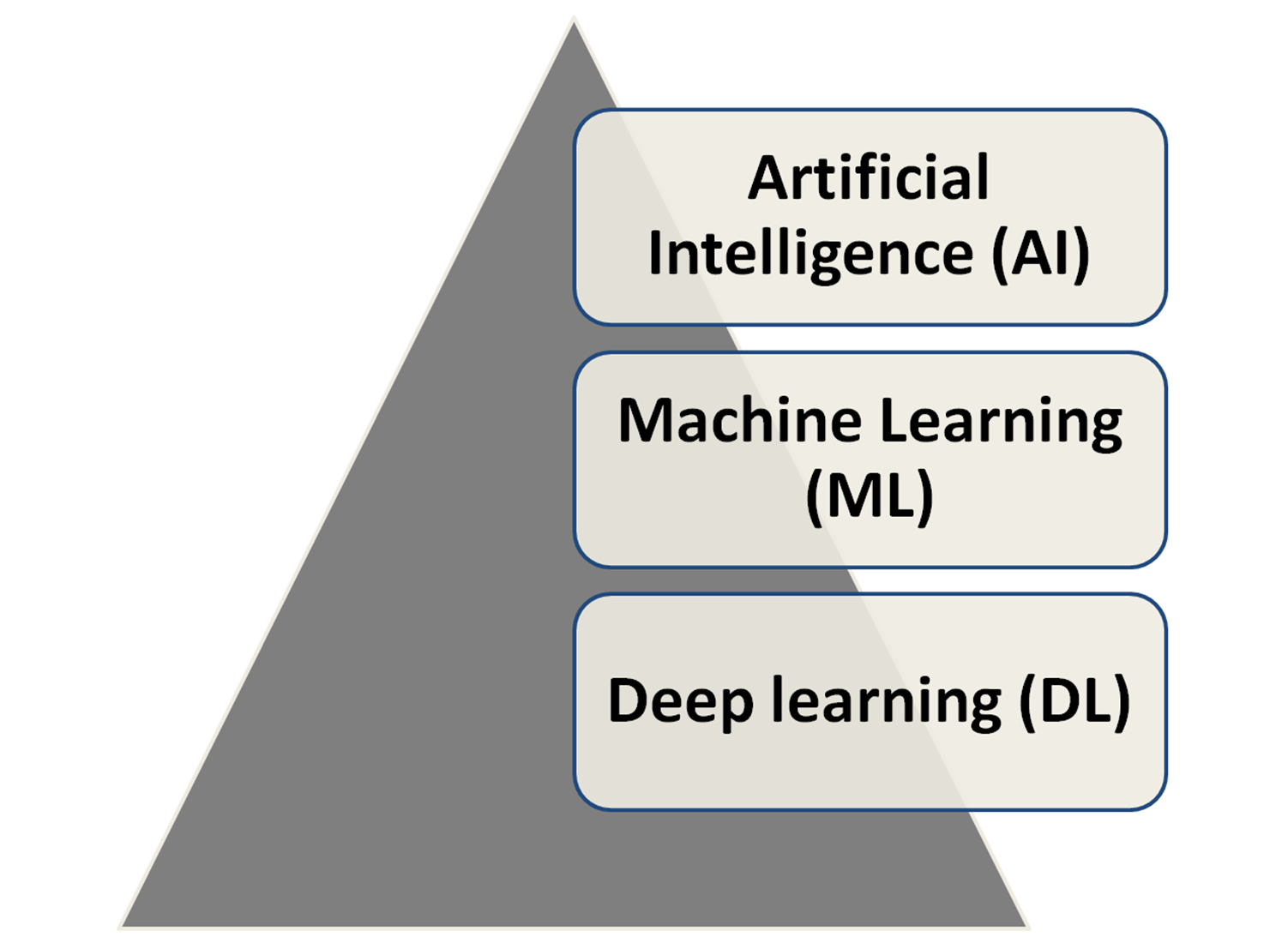## Inspiration for neural networks

Neural networks are inspired by the way the human brain works. A human brain can process huge amounts of information using data sent by human senses (especially vision). The processing is done by neurons, which work on electrical signals passing through them and applying flip-flop logic, like opening and closing of the gates for signal to transmit through. The following images shows the structure of a neuron: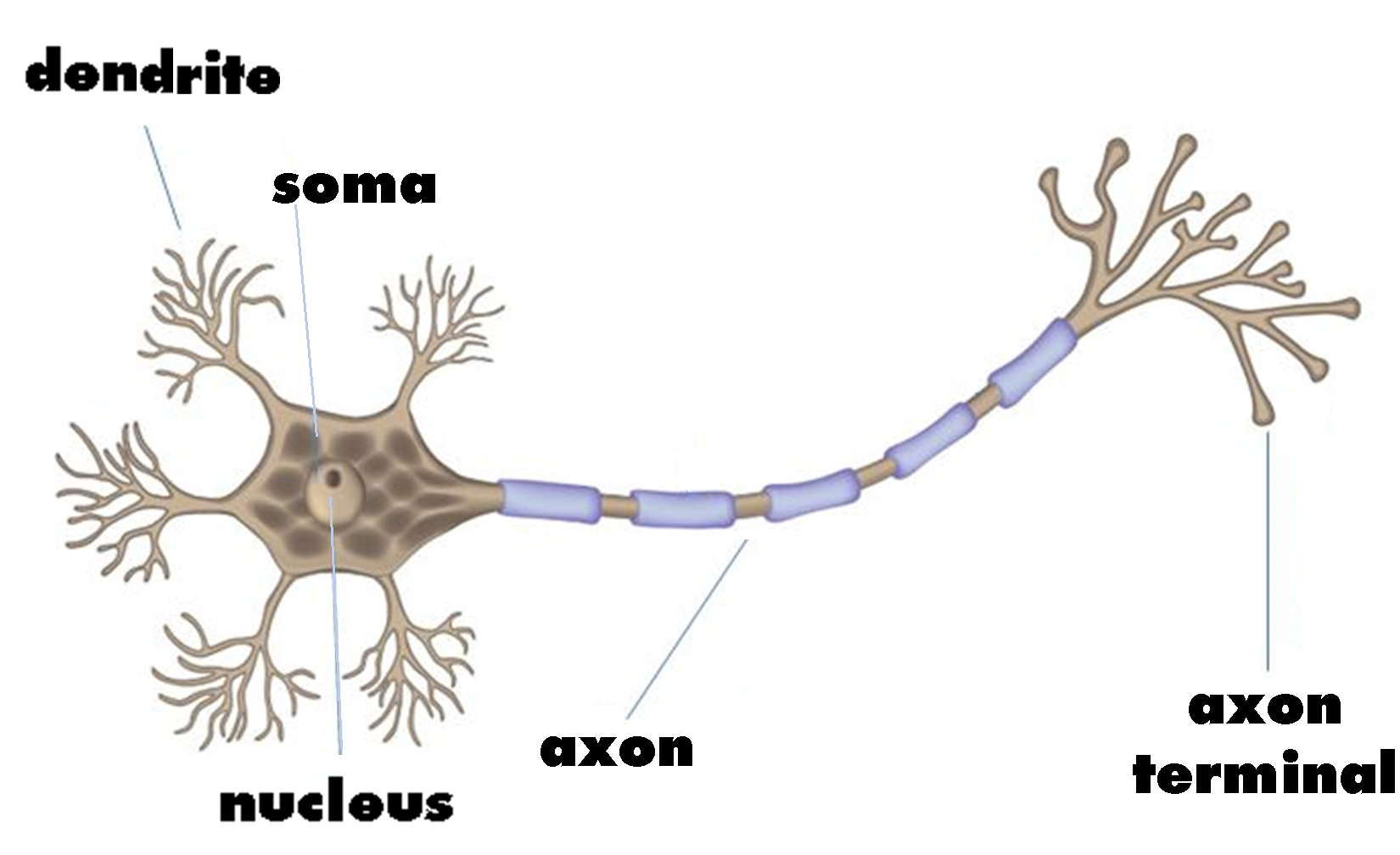The major components of each neuron are:

• Dendrites: Entry points in each neuron which take input from other neurons in the network in form of electrical impulses
• Cell Body: It generates inferences from the dendrite inputs and decides what action to take
• Axon terminals: They transmit outputs in form of electrical impulses to next neuron

Each neuron processes signals only if it exceeds a certain threshold. Neurons either fire or do not fire; it is either 0 or 1.

AI has been a domain for sci-fi movies and fiction books. ANNs within AI have been around since the 1950s, but we have made them more dominant in the past 10 years due to advances in computing architecture and performance. There have been major advancements in computer processing, leading to:

• Massive parallelism
• Distributed representation and computation
• Learning and generalization ability
• Fault tolerance
• Low energy consumption

In the domain of numerical computations and symbol manipulation, solving problems on-top of centralized architecture, modern day computers have surpassed humans to a greater extent. Where they actually lag behind with such an organizing structure is in the domains of pattern recognition, noise reduction, and optimizing. A toddler can recognize his/her mom in a huge crowd, but a computer with a centralized architecture wouldn’t be able to do the same.

This is where the biological neural network of the brain has been outperforming machines, and hence the inspiration to develop an alternative loosely held, decentralized architecture mimicking the brain.

ANNs are massively parallel computing systems consisting of an extremely large number of simple processors with many interconnections.

One of the leading global news agencies, Guardian, used big data in digitizing the archives by uploading the snapshots of all the archives they had had. However, for a user to copy the content and use it elsewhere is the limitation here. To overcome that, one can use an ANN for text pattern recognition to convert the images to text file and then to any format according to the needs of the end-users.

## How do neural networks work?

Similar to the biological neuron structure, ANNs define the neuron as a central processing unit, which performs a mathematical operation to generate one output from a set of inputs. The output of a neuron is a function of the weighted sum of the inputs plus the bias. Each neuron performs a very simple operation that involves activating if the total amount of signal received exceeds an activation threshold, as shown in the following figure: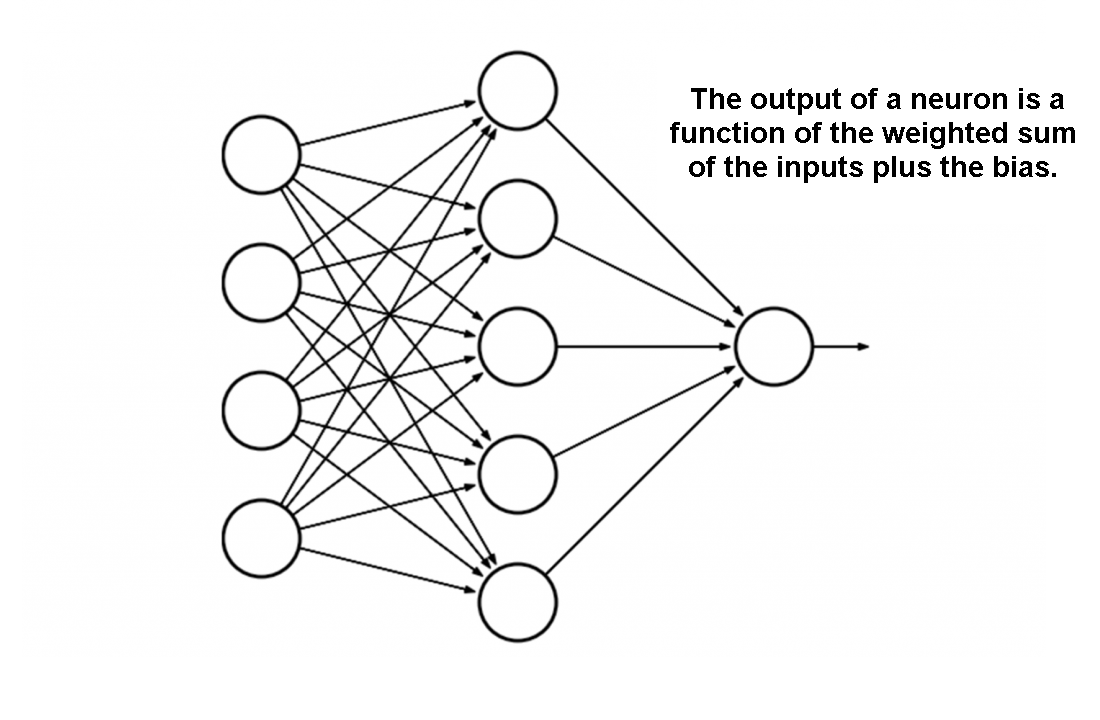The function of the entire neural network is simply the computation of the outputs of all the neurons, which is an entirely deterministic calculation. Essentially, ANN is a set of mathematical function approximations. We would now be introducing new terminology associated with ANNs:

• Input layer
• Hidden layer
• Output layer
• Weights
• Bias
• Activation functions

## Layered approach

Any neural network processing a framework has the following architecture: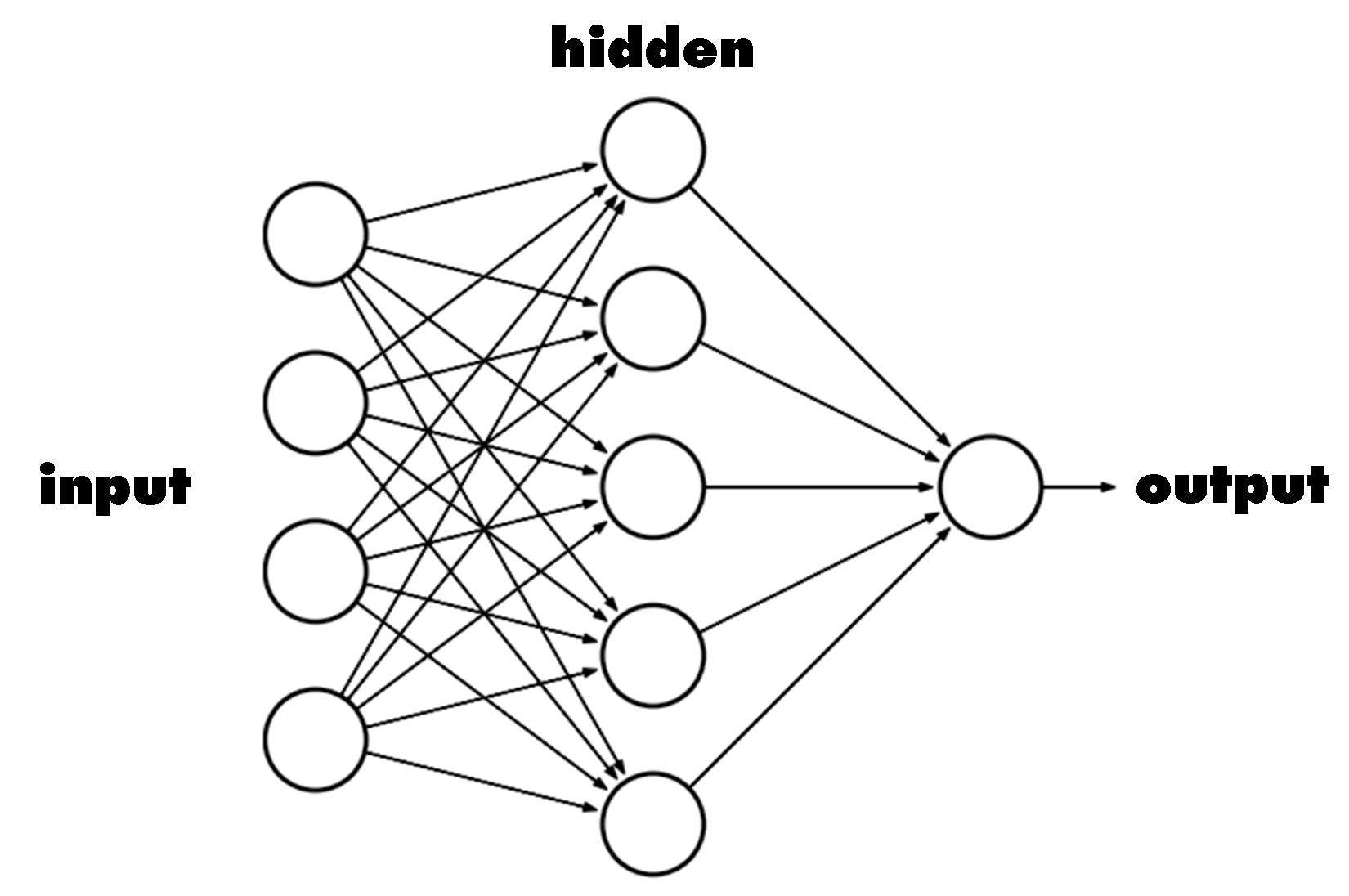There is a set of inputs, a processor, and a set of outputs. This layered approach is also followed in neural networks. The inputs form the input layer, the middle layer(s) which performs the processing is called the hidden layer(s), and the output(s) forms the output layer.

Our neural network architectures are also based on the same principle. The hidden layer has the magic to convert the input to the desired output. The understanding of the hidden layer requires knowledge of weights, bias, and activation functions, which is our next topic of discussion.

## Weights and biases

Weights in an ANN are the most important factor in converting an input to impact the output. This is similar to slope in linear regression, where a weight is multiplied to the input to add up to form the output. Weights are numerical parameters which determine how strongly each of the neurons affects the other.

For a typical neuron, if the inputs are x1, x2, and x3, then the synaptic weights to be applied to them are denoted as w1, w2, and w3.

Output is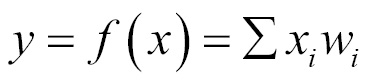where i is 1 to the number of inputs.

Simply, this is a matrix multiplication to arrive at the weighted sum.

Bias is like the intercept added in a linear equation. It is an additional parameter which is used to adjust the output along with the weighted sum of the inputs to the neuron.

The processing done by a neuron is thus denoted as :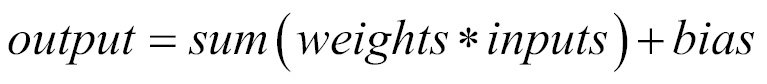A function is applied on this output and is called an activation function. The input of the next layer is the output of the neurons in the previous layer, as shown in the following image: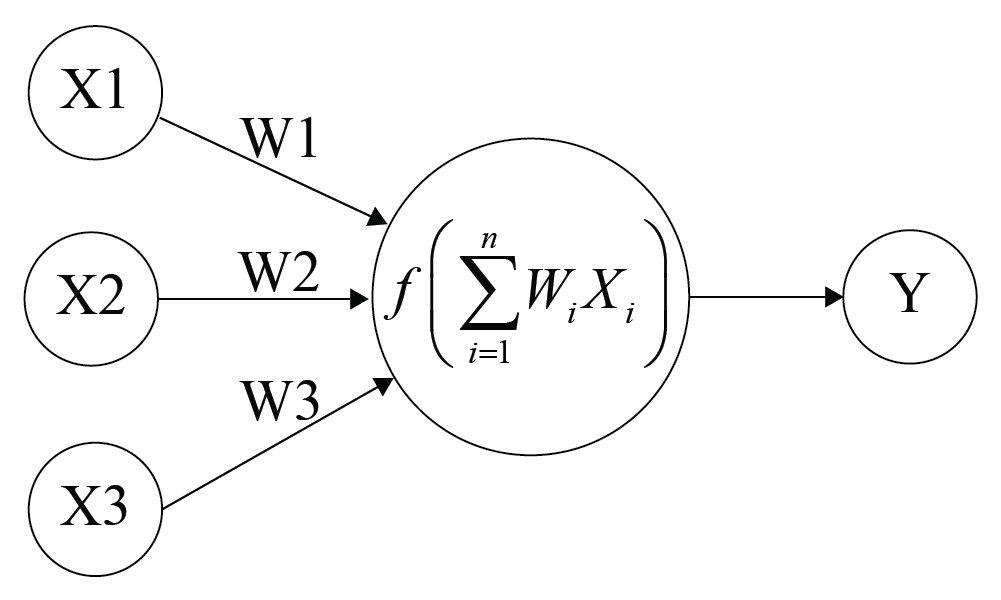## Training neural networks

Training is the act of presenting the network with some sample data and modifying the weights to better approximate the desired function.

There are two main types of training: supervised learning and unsupervised learning.

### Supervised learning

We supply the neural network with inputs and the desired outputs. Response of the network to the inputs is measured. The weights are modified to reduce the difference between the actual and desired outputs.

### Unsupervised learning

We only supply inputs. The neural network adjusts its own weights, so that similar inputs cause similar outputs. The network identifies the patterns and differences in the inputs without any external assistance.

## Epoch

One iteration or pass through the process of providing the network with an input and updating the network's weights is called an epoch. It is a full run of feed-forward and backpropagation for update of weights. It is also one full read through of the entire dataset.

Typically, many epochs, in the order of tens of thousands at times, are required to train the neural network efficiently. We will see more about epochs in the forthcoming chapters.

## Activation functions

The abstraction of the processing of neural networks is mainly achieved through the activation functions. An activation function is a mathematical function which converts the input to an output, and adds the magic of neural network processing. Without activation functions, the working of neural networks will be like linear functions. A linear function is one where the output is directly proportional to input, for example: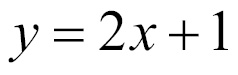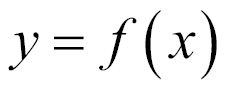A linear function is a polynomial of one degree. Simply, it is a straight line without any curves.

However, most of the problems the neural networks try to solve are nonlinear and complex in nature. To achieve the nonlinearity, the activation functions are used. Nonlinear functions are high degree polynomial functions, for example: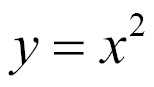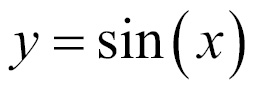The graph of a nonlinear function is curved and adds the complexity factor.

Activation functions give the nonlinearity property to neural networks and make them true universal function approximators.

## Different activation functions

There are many activation functions available for a neural network to use. We shall see a few of them here.

### Linear function

The simplest activation function, one that is commonly used for the output layer activation function in neural network problems, is the linear activation function represented by the following formula: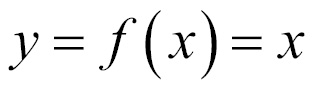The output is same as the input and the function is defined in the range (-infinity, +infinity). In the following figure, a linear activation function is shown: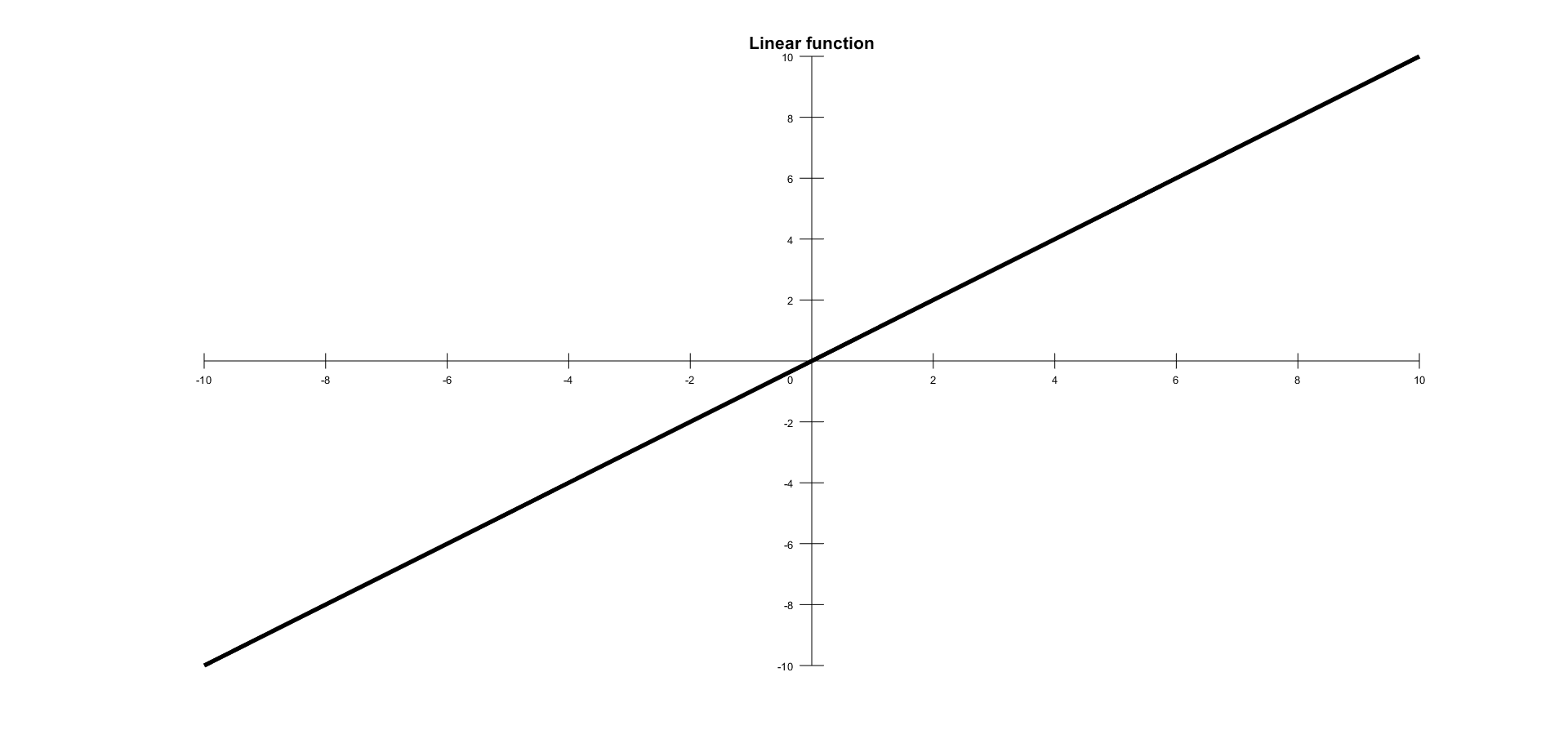### Unit step activation function

A unit step activation function is a much-used feature in neural networks. The output assumes value 0 for negative argument and 1 for positive argument. The function is as follows: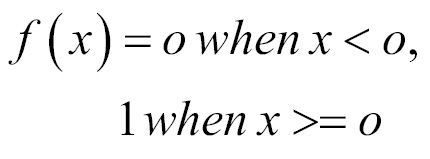The range is between (0,1) and the output is binary in nature. These types of activation functions are useful for binary schemes. When we want to classify an input model in one of two groups, we can use a binary compiler with a unit step activation function. A unit step activation function is shown in the following figure: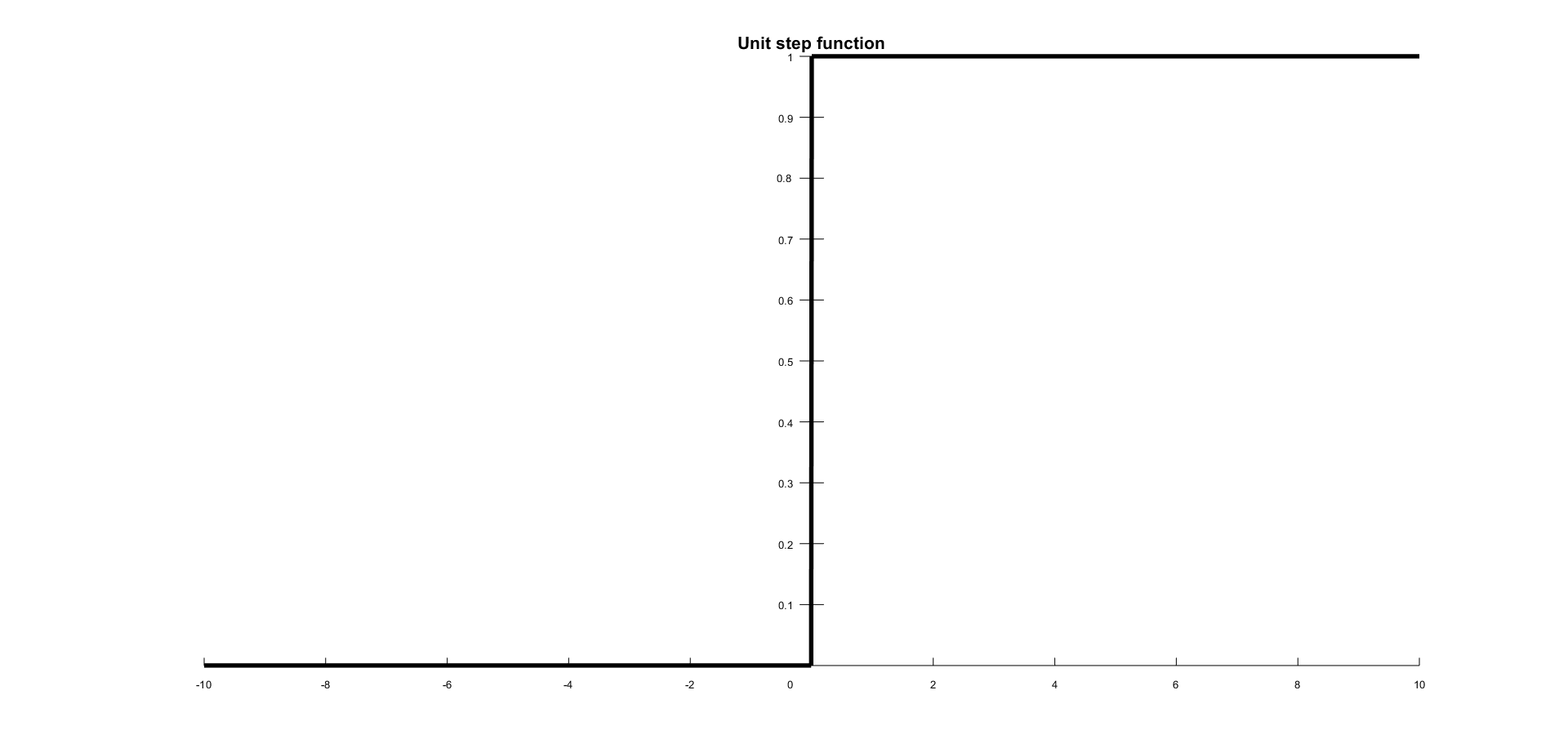### Sigmoid

The sigmoid function is a mathematical function that produces a sigmoidal curve; a characteristic curve for its S shape. This is the earliest and often used activation function. This squashes the input to any value between 0 and 1, and makes the model logistic in nature. This function refers to a special case of logistic function defined by the following formula: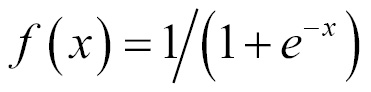In the following figure is shown a sigmoid curve with an S shape: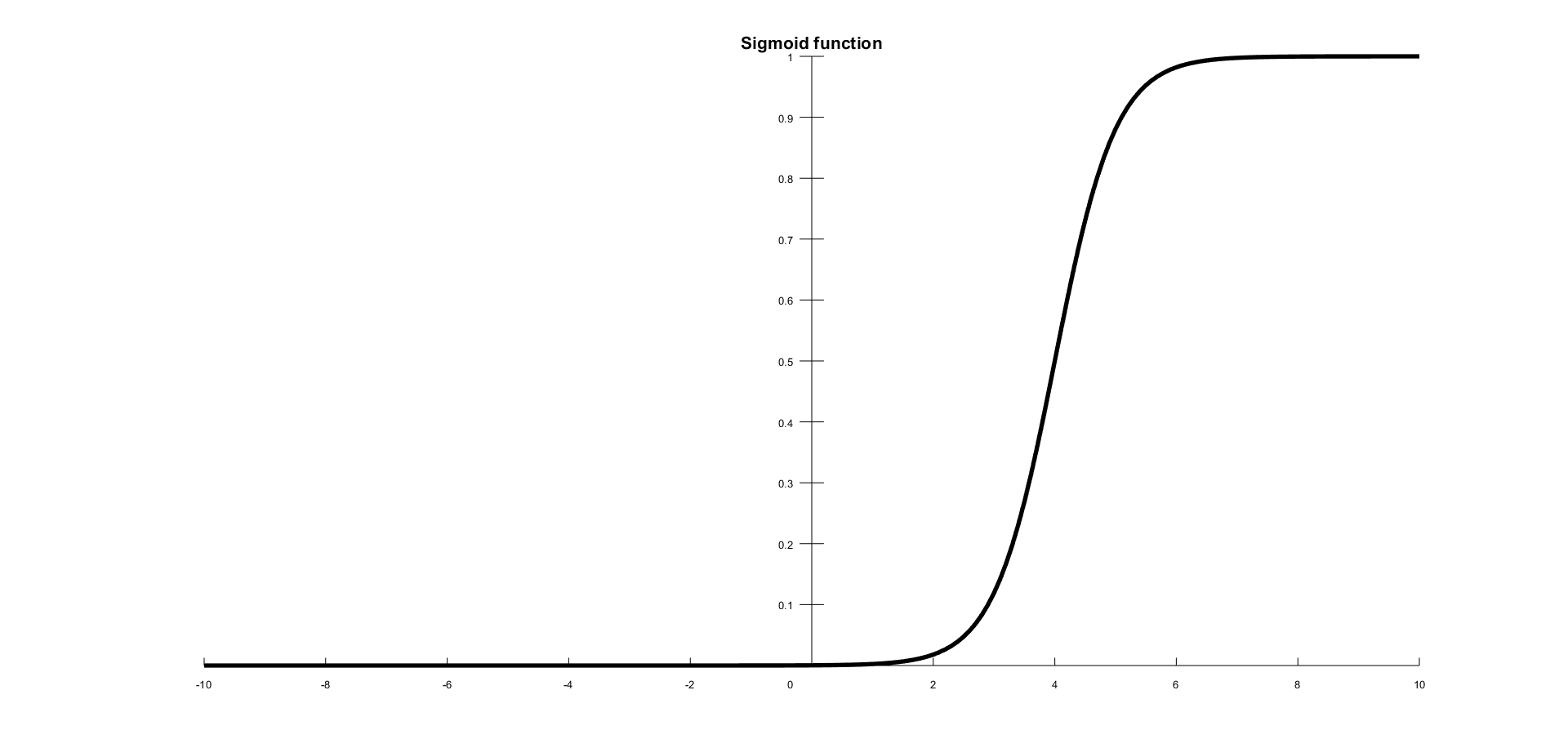### Hyperbolic tangent

Another very popular and widely used activation feature is the tanh function. If you look at the figure that follows, you can notice that it looks very similar to sigmoid; in fact, it is a scaled sigmoid function. This is a nonlinear function, defined in the range of values (-1, 1), so you need not worry about activations blowing up. One thing to clarify is that the gradient is stronger for tanh than sigmoid (the derivatives are more steep). Deciding between sigmoid and tanh will depend on your gradient strength requirement. Like the sigmoid, tanh also has the missing slope problem. The function is defined by the following formula: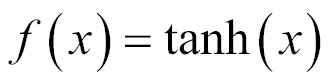In the following figure is shown a hyberbolic tangent activation function: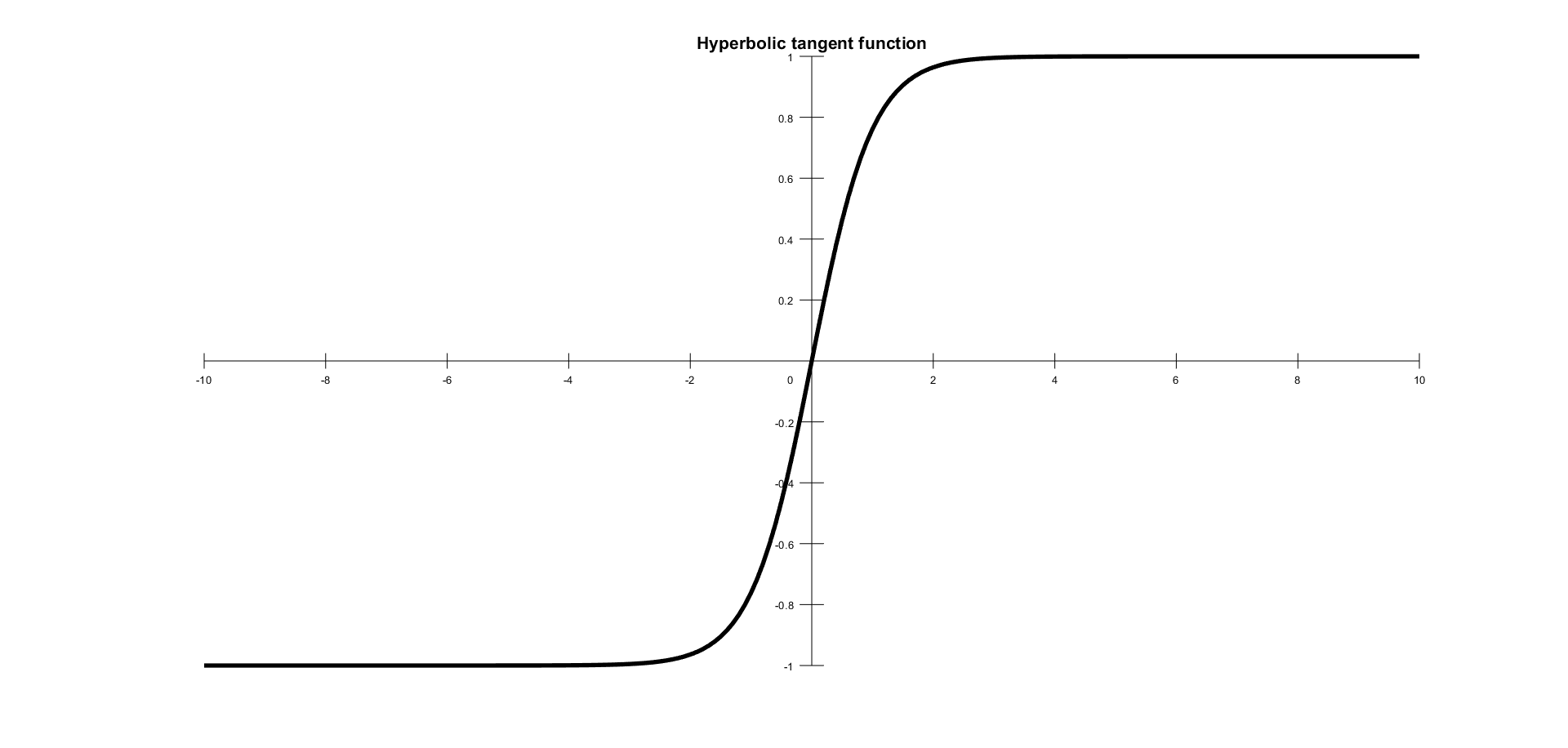This looks very similar to sigmoid; in fact, it is a scaled sigmoid function.

### Rectified Linear Unit

Rectified Linear Unit (ReLU) is the most used activation function since 2015. It is a simple condition and has advantages over the other functions. The function is defined by the following formula: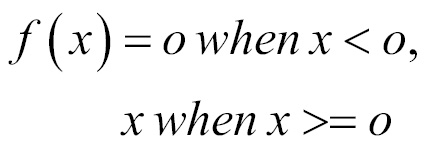In the following figure is shown a ReLU activation function: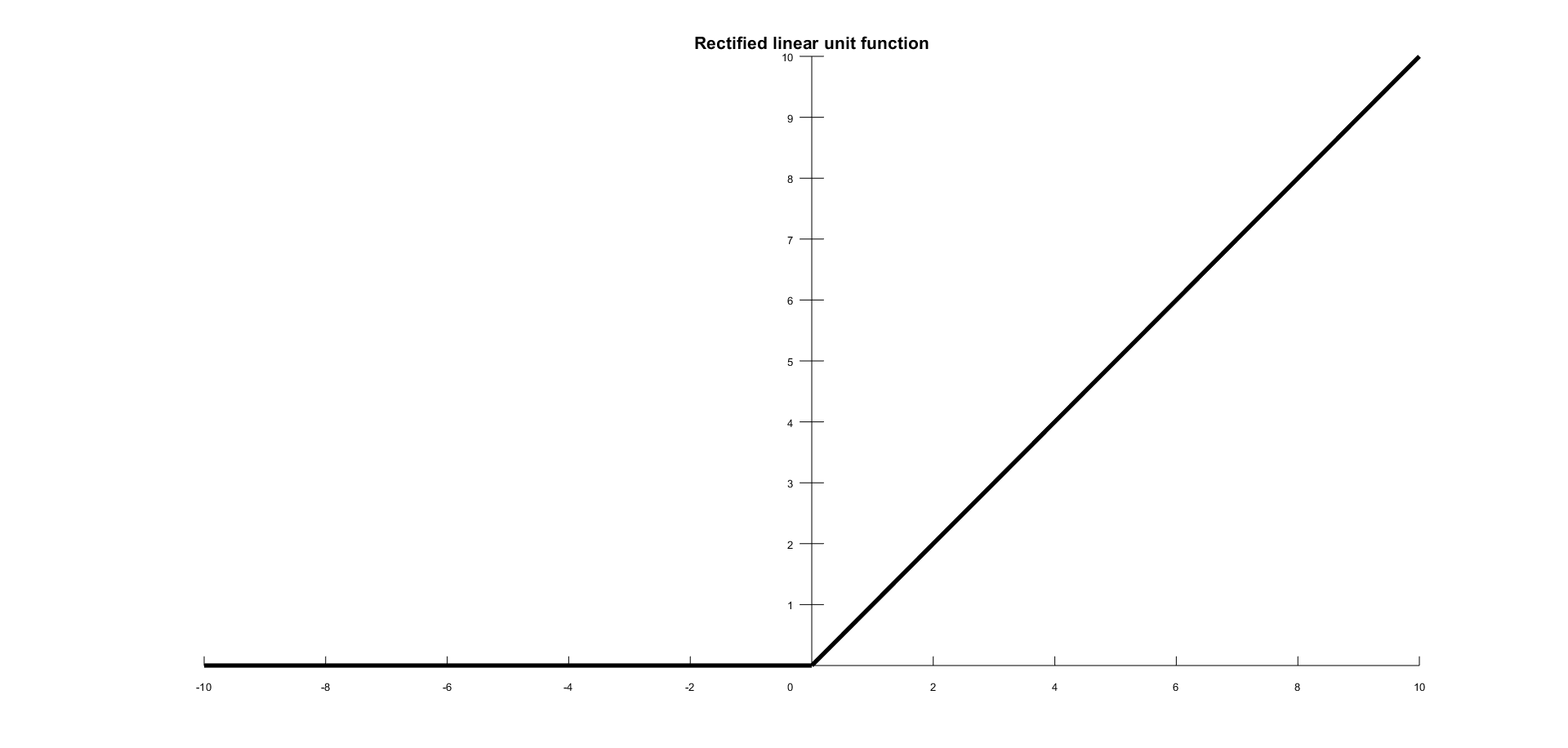The range of output is between 0 and infinity. ReLU finds applications in computer vision and speech recognition using deep neural nets. There are various other activation functions as well, but we have covered the most important ones here.

## Which activation functions to use?

Given that neural networks are to support nonlinearity and more complexity, the activation function to be used has to be robust enough to have the following:

• It should be differential; we will see why we need differentiation in backpropagation. It should not cause gradients to vanish.
• It should be simple and fast in processing.
• It should not be zero centered.

The sigmoid is the most used activation function, but it suffers from the following setbacks:

• Since it uses logistic model, the computations are time consuming and complex
• It cause gradients to vanish and no signals pass through the neurons at some point of time
• It is slow in convergence
• It is not zero centered

These drawbacks are solved by ReLU. ReLU is simple and is faster to process. It does not have the vanishing gradient problem and has shown vast improvements compared to the sigmoid and tanh functions. ReLU is the most preferred activation function for neural networks and DL problems.

ReLU is used for hidden layers, while the output layer can use a `softmax` function for logistic problems and a linear function of regression problems.

## Perceptron and multilayer architectures

A perceptron is a single neuron that classifies a set of inputs into one of two categories (usually 1 or -1). If the inputs are in the form of a grid, a perceptron can be used to recognize visual images of shapes. The perceptron usually uses a step function, which returns 1 if the weighted sum of the inputs exceeds a threshold, and 0 otherwise.

When layers of perceptron are combined together, they form a multilayer architecture, and this gives the required complexity of the neural network processing. Multi-Layer Perceptrons (MLPs) are the most widely used architecture for neural networks.

## Forward and backpropagation

The processing from input layer to hidden layer(s) and then to the output layer is called forward propagation. The sum(input*weights)+bias is applied at each layer and then the activation function value is propagated to the next layer. The next layer can be another hidden layer or the output layer. The construction of neural networks uses large number of hidden layers to give rise to Deep Neural Network (DNN).

Once the output is arrived at, at the last layer (the output layer), we compute the error (the predicted output minus the original output). This error is required to correct the weights and biases used in forward propagation. Here is where the derivative function is used. The amount of weight that has to be changed is determined by gradient descent.

The backpropagation process uses the partial derivative of each neuron's activation function to identify the slope (or gradient) in the direction of each of the incoming weights. The gradient suggests how steeply the error will be reduced or increased for a change in the weight. The backpropagation keeps changing the weights until there is greatest reduction in errors by an amount known as the learning rate.

Learning rate is a scalar parameter, analogous to step size in numerical integration, used to set the rate of adjustments to reduce the errors faster. Learning rate is used in backpropagation during adjustment of weights and bias.

More the learning rate, the faster the algorithm will reduce the errors and faster will be the training process: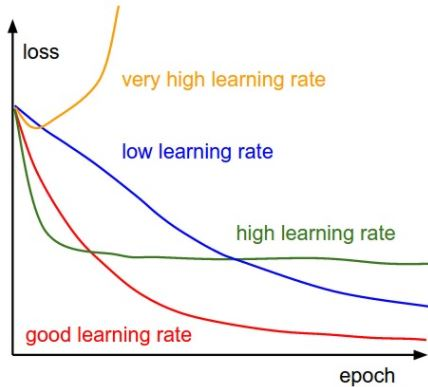## Step-by-step illustration of a neuralnet and an activation function

We shall take a step-by-step approach to understand the forward and reverse pass with a single hidden layer. The input layer has one neuron and the output will solve a binary classification problem (predict 0 or 1). In the following figure is shown a forward and reverse pass with a single hidden layer: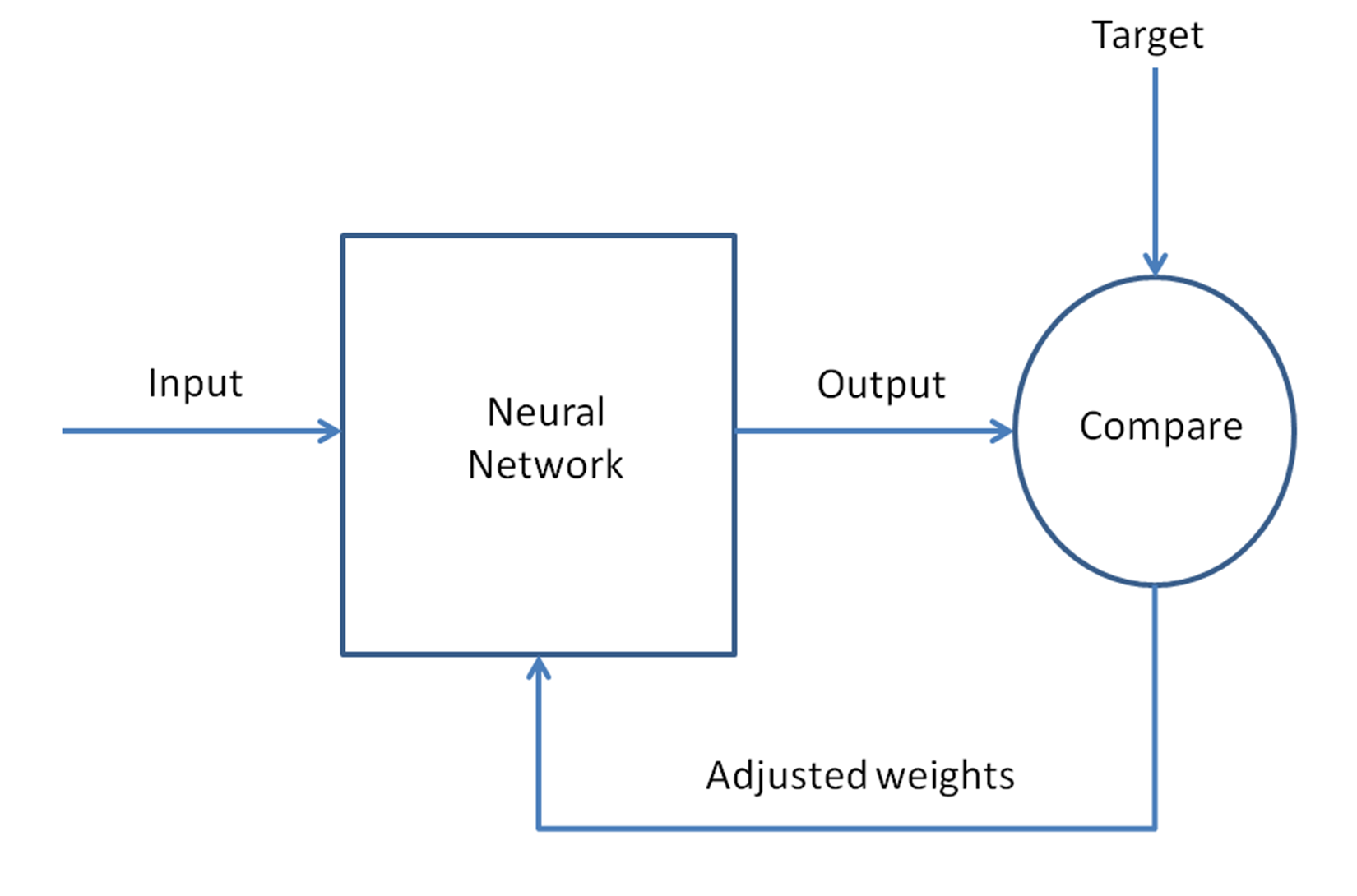Next, let us analyze in detail, step by step, all the operations to be done for network training:

1. Take the input as a matrix.
2. Initialize the weights and biases with random values. This is one time and we will keep updating these with the error propagation process.
3. Repeat the steps 4 to 9 for each training pattern (presented in random order), until the error is minimized.
4. Apply the inputs to the network.
5. Calculate the output for every neuron from the input layer, through the hidden layer(s), to the output layer.
6. Calculate the error at the outputs: actual minus predicted.

1. Use the output error to compute error signals for previous layers. The partial derivative of the activation function is used to compute the error signals.
2. Use the error signals to compute weight adjustments.

Steps 4 and 5 are forward propagation and steps 6 through 9 are backpropagation.

The learning rate is the amount that weights are updated is controlled by a configuration parameter.

The complete pass back and forth is called a training cycle or epoch. The updated weights and biases are used in the next cycle. We keep recursively training until the error is very minimal.

We shall cover more about the forward and backpropagation in detail throughout this book.

## Feed-forward and feedback networks

The flow of the signals in neural networks can be either in only one direction or in recurrence. In the first case, we call the neural network architecture feed-forward, since the input signals are fed into the input layer, then, after being processed, they are forwarded to the next layer, just as shown in the following figure. MLPs and radial basis functions are also good examples of feed-forward networks. In the following figure is shown an MLPs architecture: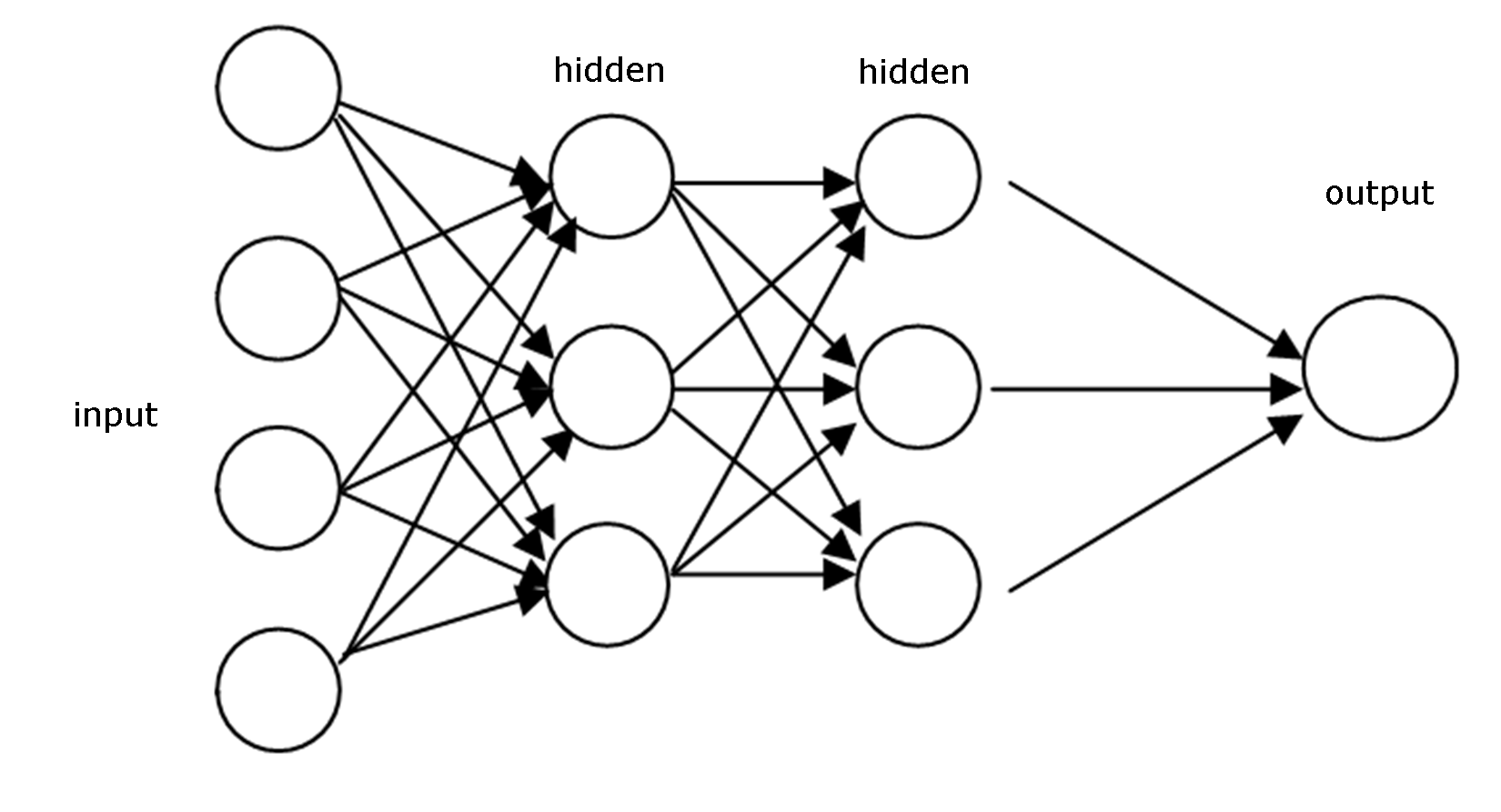When the neural network has some kind of internal recurrence, meaning that the signals are fed back to a neuron or layer that has already received and processed that signal, the network is of the type feedback, as shown in the following image: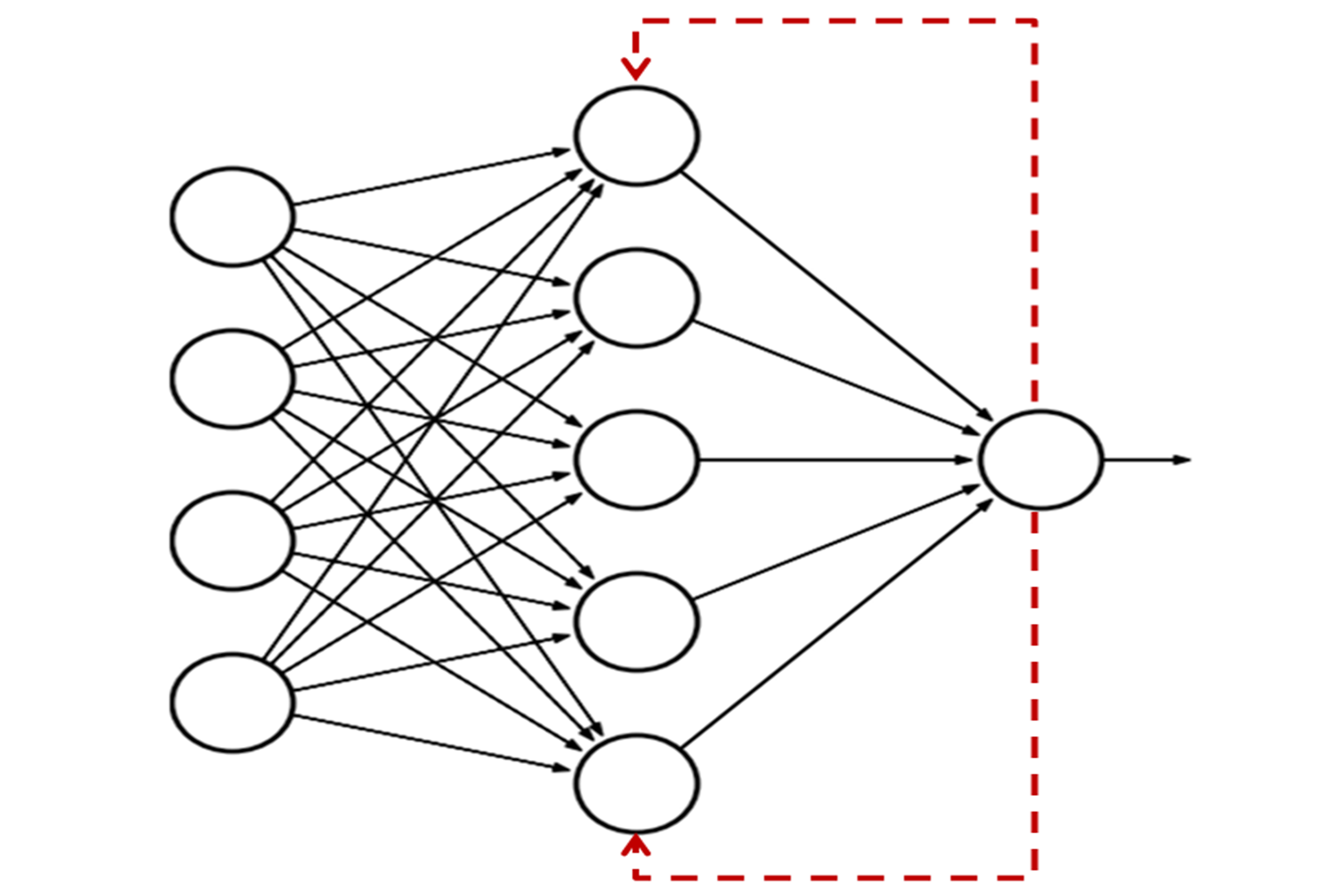The special reason to add recurrence in a network is the production of a dynamic behavior, particularly when the network addresses problems involving time series or pattern recognition, that require an internal memory to reinforce the learning process. However, such networks are particularly difficult to train, eventually failing to learn. Most of the feedback networks are single layer, such as the Elman and Hopfield networks, but it is possible to build a recurrent multilayer network, such as echo and recurrent MLP networks.

Gradient descent is an iterative approach for error correction in any learning model. For neural networks during backpropagation, the process of iterating the update of weights and biases with the error times derivative of the activation function is the gradient descent approach. The steepest descent step size is replaced by a similar size from the previous step. Gradient is basically defined as the slope of the curve and is the derivative of the activation function: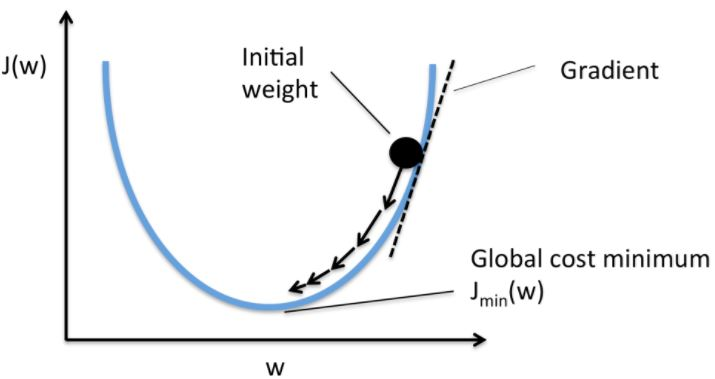The objective of deriving gradient descent at each step is to find the global cost minimum, where the error is the lowest. And this is where the model has a good fit for the data and predictions are more accurate.

Gradient descent can be performed either for the full batch or stochastic. In full batch gradient descent, the gradient is computed for the full training dataset, whereas Stochastic Gradient Descent (SGD) takes a single sample and performs gradient calculation. It can also take mini-batches and perform the calculations. One advantage of SGD is faster computation of gradients.

## Taxonomy of neural networks

The basic foundation for ANNs is the same, but various neural network models have been designed during its evolution. The following are a few of the ANN models:

• Adaptive Linear Element (ADALINE), is a simple perceptron which can solve only linear problems. Each neuron takes the weighted linear sum of the inputs and passes it to a bi-polar function, which either produces a +1 or -1 depending on the sum. The function checks the sum of the inputs passed and if the net is >= 0, it is +1, else it is -1.
• Perceptrons are single layer neural networks (single neuron or unit), where the input is multidimensional (vector) and the output is a function on the weight sum of the inputs.
• Radial basis function network is an ANN where a radial basis function is used as an activation function. The network output is a linear combination of radial basis functions of the inputs and some neuron parameters.
• Feed-forward is the simplest form of neural networks. The data is processed across layers without any loops are cycles. We will study the following feed- forward networks in this book:
• Autoencoder
• Probabilistic
• Time delay
• Covolutional
• Recurrent Neural Networks (RNNs), unlike feed-forward networks, propagate data forward and also backwards from later processing stages to earlier stages. The following are the types of RNNs; we shall study them in our later chapters:
• Hopfield networks
• Boltzmann machine
• Self Organizing Maps (SOMs)
• Bidirectional Associative Memory (BAM)
• Long Short Term Memory (LSTM)

The following images depict (a) Recurrent neural network and (b) Forward neural network: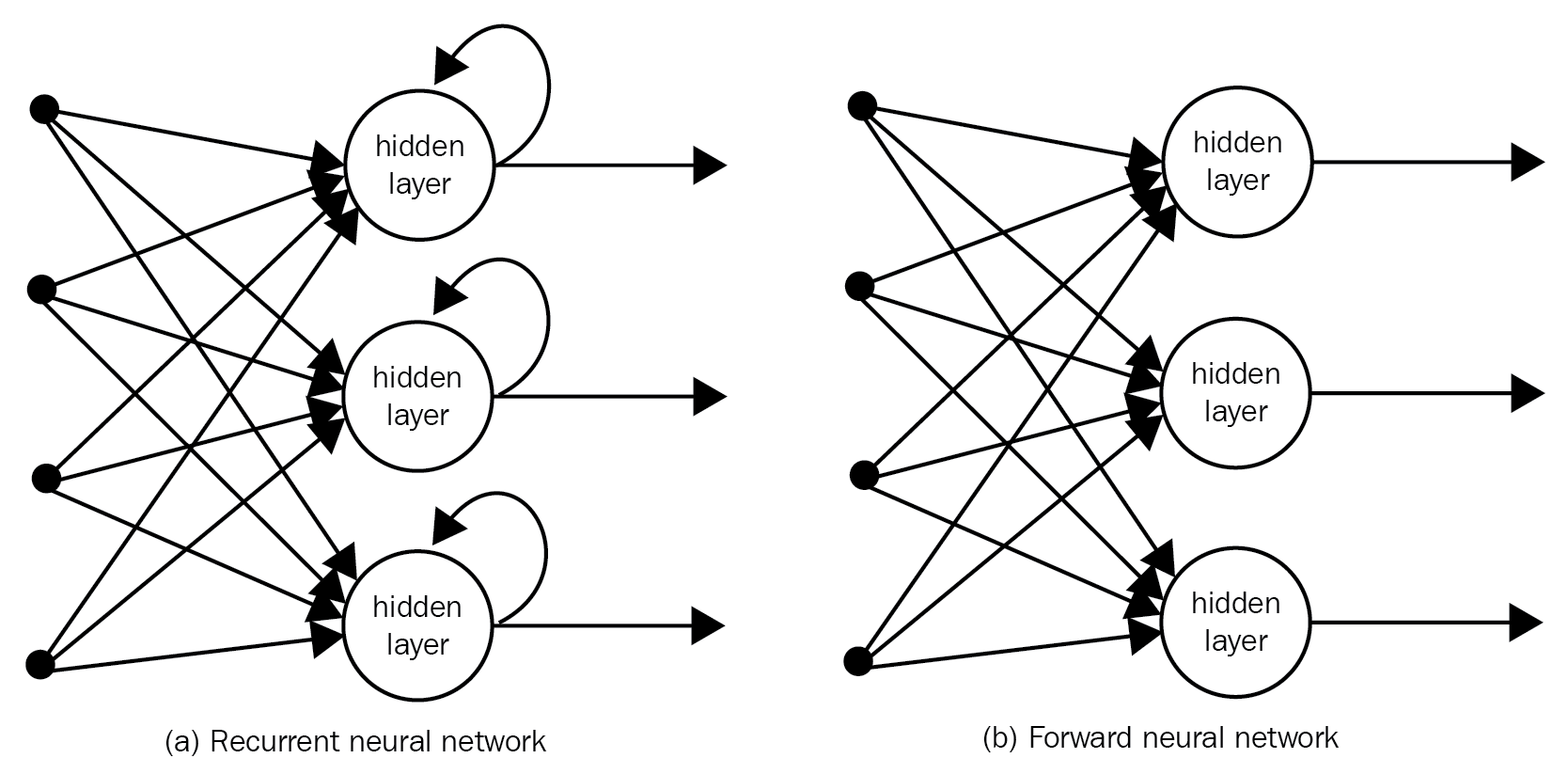## Simple example using R neural net library - neuralnet()

Consider a simple dataset of a square of numbers, which will be used to train a `neuralnet` function in R and then test the accuracy of the built neural network:

 INPUT OUTPUT `0` `0` `1` `1` `2` `4` `3` `9` `4` `16` `5` `25` `6` `36` `7` `49` `8` `64` `9` `81` `10` `100`

Our objective is to set up the weights and bias so that the model can do what is being done here. The output needs to be modeled on a function of input and the function can be used in future to determine the output based on an input:

```#########################################################################
###Chapter 1 - Introduction to Neural Networks - using R ################
###Simple R program to build, train and test neural Networks#############
#########################################################################

#Choose the libraries to use
library("neuralnet")

#Set working directory for the training data
setwd("C:/R")
getwd()

mydata
attach(mydata)
names(mydata)

#Train the model based on output from input
model=neuralnet(formula = Output~Input,
data = mydata,
hidden=10,
threshold=0.01 )
print(model)

#Lets plot and see the layers
plot(model)

#Check the data - actual and predicted
final_output=cbind (Input, Output,
as.data.frame(model\$net.result) )
colnames(final_output) = c("Input", "Expected Output",
"Neural Net Output" )
print(final_output)
#########################################################################```

### Let us go through the code line-by-line

To understand all the steps in the code just proposed, we will look at them in detail. Do not worry if a few steps seem unclear at this time, you will be able to look into it in the following examples. First, the code snippet will be shown, and the explanation will follow:

`library("neuralnet")`

The line in R includes the library `neuralnet()` in our program. `neuralnet()` is part of Comprehensive R Archive Network (CRAN), which contains numerous R libraries for various applications.

```mydata=read.csv('Squares.csv',sep=",",header=TRUE)
mydata
attach(mydata)
names(mydata)```

This reads the CSV file with separator `,`(comma), and header is the first line in the file. `names()` would display the header of the file.

```model=neuralnet(formula = Output~Input,
data = mydata,
hidden=10,
threshold=0.01 )```

The training of the output with respect to the input happens here. The `neuralnet()` library is passed the output and input column names (`ouput~input`), the dataset to be used, the number of neurons in the hidden layer, and the stopping criteria (`threshold`).

A brief description of the `neuralnet` package, extracted from the official documentation, is shown in the following table:

 neuralnet-package: Description: Training of neural networks using the backpropagation, resilient backpropagation with (Riedmiller, 1994) or without weight backtracking (Riedmiller, 1993), or the modified globally convergent version by Anastasiadis et al. (2005). The package allows flexible settings through custom-choice of error and activation function. Furthermore, the calculation of generalized weights (Intrator O & Intrator N, 1993) is implemented. Details: Package: `neuralnet`Type: PackageVersion: 1.33Date: 2016-08-05License: GPL (>=2) Authors: Stefan Fritsch, Frauke Guenther (email: `guenther@leibniz-bips.de`)Maintainer: Frauke Guenther (email: `guenther@leibniz-bips.de`) Usage: `neuralnet(formula, data, hidden = 1, threshold = 0.01, stepmax = 1e+05, rep = 1, startweights = NULL, learningrate.limit = NULL, learningrate.factor = list(minus = 0.5, plus = 1.2), learningrate=NULL, lifesign = "none", lifesign.step = 1000, algorithm = "rprop+", err.fct = "sse", act.fct = "logistic", linear.output = TRUE, exclude = NULL,``constant.weights = NULL, likelihood = FALSE)` Meaning of the arguments: `formula`: A symbolic description of the model to be fitted.`data`: A dataframe containing the variables specified in formula.`hidden`: A vector of integers specifying the number of hidden neurons (vertices) in each layer.`threshold`: A numeric value specifying the threshold for the partial derivatives of the error function as stopping criteria.`stepmax`: The maximum steps for the training of the neural network. Reaching this maximum leads to a stop of the neural network's training process.`rep`: The number of repetitions for the neural network's training.`startweights`: A vector containing starting values for the weights. The weights will not be randomly initialized.`learningrate.limit`: A vector or a list containing the lowest and highest limit for the learning rate. Used only for `RPROP` and `GRPROP`.`learningrate.factor`: A vector or a list containing the multiplication factors for the upper and lower learning rate, used only for `RPROP` and `GRPROP`.`learningrate`: A numeric value specifying the learning rate used by traditional backpropagation. Used only for traditional backpropagation.`lifesign`: A string specifying how much the function will print during the calculation of the neural network-`'none'`, `'minimal'`, or `'full'`.`lifesign.step`: An integer specifying the step size to print the minimal threshold in full lifesign mode.`algorithm`: A string containing the algorithm type to calculate the neural network.`err.fct`: A differentiable function that is used for the calculation of the error.`act.fct`: A differentiable function that is used for smoothing the result of the cross product of the covariate or neurons and the weights.`linear.output`: Logical. If `act.fct` should not be applied to the output neurons set linear output to `TRUE`, otherwise to `FALSE`.`exclude`: A vector or a matrix specifying the weights that are excluded from the calculation.`constant.weights`: A vector specifying the values of the weights that are excluded from the training process and treated as fix.`likelihood`: Logical. If the error function is equal to the negative log-likelihood function, the information criteria AIC and BIC will be calculated. Furthermore the usage of confidence. interval is meaningful.

After giving a brief glimpse into the package documentation, let's review the remaining lines of the proposed code sample:

` print(model)`

This command prints the model that has just been generated, as follows:

```\$result.matrix
1
error                          0.001094100442
reached.threshold              0.009942937680
steps                      34563.000000000000
Intercept.to.1layhid1         12.859227998180
Input.to.1layhid1             -1.267870997079
Intercept.to.1layhid2         11.352189417430
Input.to.1layhid2             -2.185293148851
Intercept.to.1layhid3          9.108325110066
Input.to.1layhid3             -2.242001064132
Intercept.to.1layhid4        -12.895335140784
Input.to.1layhid4              1.334791491801
Intercept.to.1layhid5         -2.764125889399
Input.to.1layhid5              1.037696638808
Intercept.to.1layhid6         -7.891447011323
Input.to.1layhid6              1.168603081208
Intercept.to.1layhid7         -9.305272978434
Input.to.1layhid7              1.183154841948
Intercept.to.1layhid8         -5.056059256828
Input.to.1layhid8              0.939818815422
Intercept.to.1layhid9         -0.716095585596
Input.to.1layhid9             -0.199246231047
Intercept.to.1layhid10        10.041789457410
Input.to.1layhid10            -0.971900813630
Intercept.to.Output           15.279512257145
1layhid.1.to.Output          -10.701406269616
1layhid.2.to.Output           -3.225793088326
1layhid.3.to.Output           -2.935972228783
1layhid.4.to.Output           35.957437333162
1layhid.5.to.Output           16.897986621510
1layhid.6.to.Output           19.159646982676
1layhid.7.to.Output           20.437748965610
1layhid.8.to.Output           16.049490298968
1layhid.9.to.Output           16.328504039013
1layhid.10.to.Output          -4.900353775268```

Let's go back to the code analysis:

`plot(model)`

This preceding command plots the neural network for us, as follows: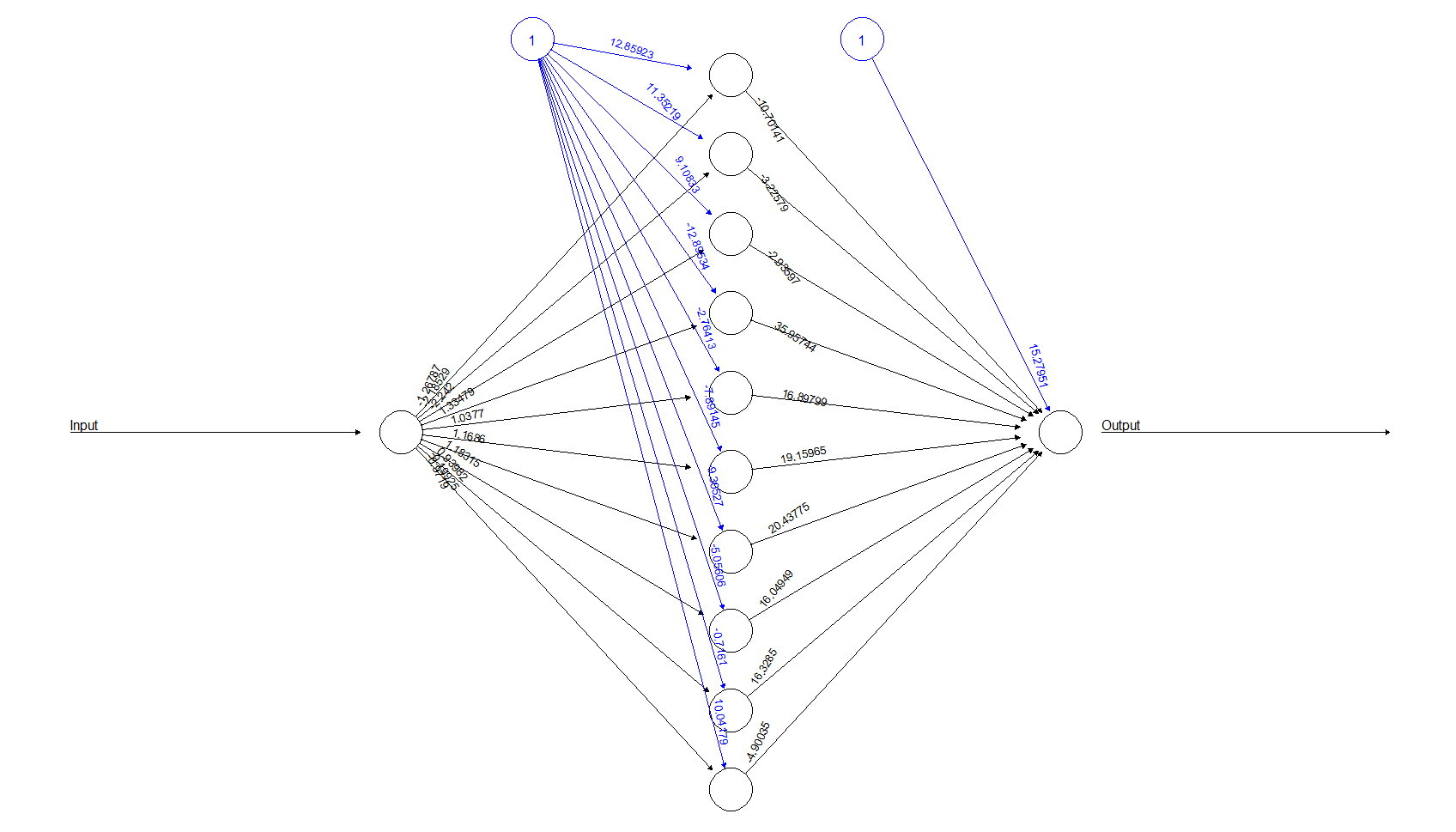```final_output=cbind (Input, Output,
as.data.frame(model\$net.result) )
colnames(final_output) = c("Input", "Expected Output",
"Neural Net Output" )
print(final_output)```

This preceding code prints the final output, comparing the output predicted and actual as:

```> print(final_output)
Input Expected Output Neural Net Output
1    0               0     -0.0108685813
2    1               1      1.0277796553
3    2               4      3.9699671691
4    3               9      9.0173879001
5    4              16     15.9950295615
6    5              25     25.0033272826
7    6              36     35.9947137155
8    7              49     49.0046689369
9    8              64     63.9972090104
10   9              81     81.0008391011
11  10             100     99.9997950184 ```

## Implementation using nnet() library

To improve our practice with the `nnet` library, we look at another example. This time we will use the data collected at a restaurant through customer interviews. The customers were asked to give a score to the following aspects: service, ambience, and food. They were also asked whether they would leave the tip on the basis of these scores. In this case, the number of inputs is `2` and the output is a categorical value (`Tip=1` and `No-tip=0`).

The input file to be used is shown in the following table:

 No CustomerWillTip Service Ambience Food TipOrNo `1` `1` `4` `4` `5` `Tip` `2` `1` `6` `4` `4` `Tip` `3` `1` `5` `2` `4` `Tip` `4` `1` `6` `5` `5` `Tip` `5` `1` `6` `3` `4` `Tip` `6` `1` `3` `4` `5` `Tip` `7` `1` `5` `5` `5` `Tip` `8` `1` `5` `4` `4` `Tip` `9` `1` `7` `6` `4` `Tip` `10` `1` `7` `6` `4` `Tip` `11` `1` `6` `7` `2` `Tip` `12` `1` `5` `6` `4` `Tip` `13` `1` `7` `3` `3` `Tip` `14` `1` `5` `1` `4` `Tip` `15` `1` `7` `5` `5` `Tip` `16` `0` `3` `1` `3` `No-tip` `17` `0` `4` `6` `2` `No-tip` `18` `0` `2` `5` `2` `No-tip` `19` `0` `5` `2` `4` `No-tip` `20` `0` `4` `1` `3` `No-tip` `21` `0` `3` `3` `4` `No-tip` `22` `0` `3` `4` `5` `No-tip` `23` `0` `3` `6` `3` `No-tip` `24` `0` `4` `4` `2` `No-tip` `25` `0` `6` `3` `6` `No-tip` `26` `0` `3` `6` `3` `No-tip` `27` `0` `4` `3` `2` `No-tip` `28` `0` `3` `5` `2` `No-tip` `29` `0` `5` `5` `3` `No-tip` `30` `0` `1` `3` `2` `No-tip`

This is a classification problem with three inputs and one categorical output. We will address the problem with the following code:

```########################################################################
##Chapter 1 - Introduction to Neural Networks - using R ################
###Simple R program to build, train and test neural networks ###########
### Classification based on 3 inputs and 1 categorical output ##########
########################################################################

###Choose the libraries to use
library(NeuralNetTools)
library(nnet)

###Set working directory for the training data
setwd("C:/R")
getwd()

mydata
attach(mydata)
names(mydata)

##Train the model based on output from input
model=nnet(CustomerWillTip~Service+Ambience+Food,
data=mydata,
size =5,
rang=0.1,
decay=5e-2,
maxit=5000)
print(model)
plotnet(model)
garson(model)

########################################################################```

### Let us go through the code line-by-line

To understand all the steps in the code just proposed, we will look at them in detail. First, the code snippet will be shown, and the explanation will follow.

```library(NeuralNetTools)
library(nnet)```

This includes the libraries `NeuralNetTools` and `nnet()` for our program.

```###Set working directory for the training data
setwd("C:/R")
getwd()
mydata
attach(mydata)
names(mydata)```

This sets the working directory and reads the input CSV file.

```##Train the model based on output from input
model=nnet(CustomerWillTip~Service+Ambience+Food,
data=mydata,
size =5,
rang=0.1,
decay=5e-2,
maxit=5000)
print(model)```

This calls the `nnet()` function with the arguments passed. The output is as follows. `nnet()` processes the forward and backpropagation until convergence:

```> model=nnet(CustomerWillTip~Service+Ambience+Food,data=mydata, size =5, rang=0.1, decay=5e-2, maxit=5000)
# weights:  26
initial  value 7.571002
iter  10 value 5.927044
iter  20 value 5.267425
iter  30 value 5.238099
iter  40 value 5.217199
iter  50 value 5.216688
final  value 5.216665
converged```

A brief description of the `nnet` package, extracted from the official documentation, is shown in the following table:

 nnet-package: Feed-forward neural networks and multinomial log-linear models Description: Software for feed-forward neural networks with a single hidden layer, and for multinomial log-linear models. Details: Package: `nnet` Type: Package Version: 7.3-12 Date: 2016-02-02 License: GPL-2 | GPL-3 Author(s): Brian RipleyWilliam Venables Usage: `nnet(formula, data, weights,subset, na.action, contrasts = NULL)` Meaning of the arguments: `Formula`: A formula of the form class ~ x1 + x2 + ...`data`: Dataframe from which variables specified in formula are preferentially to be taken`weights`: (Case) weights for each example; if missing, defaults to 1`subset`: An index vector specifying the cases to be used in the training sample`na.action`: A function to specify the action to be taken if NAs are found`contrasts:` A list of contrasts to be used for some or all of the factors appearing as variables in the model formula

After giving a brief glimpse into the package documentation, let's review the remaining lines of the proposed in the following code sample:

`print(model)`

This command prints the details of the `net()` as follows:

```> print(model)
a 3-5-1 network with 26 weights
inputs: Service Ambience Food
output(s): CustomerWillTip
options were - decay=0.05```

To plot the `model`, use the following command:

`plotnet(model)`

The plot of the `model` is as follows; there are five nodes in the single hidden layer: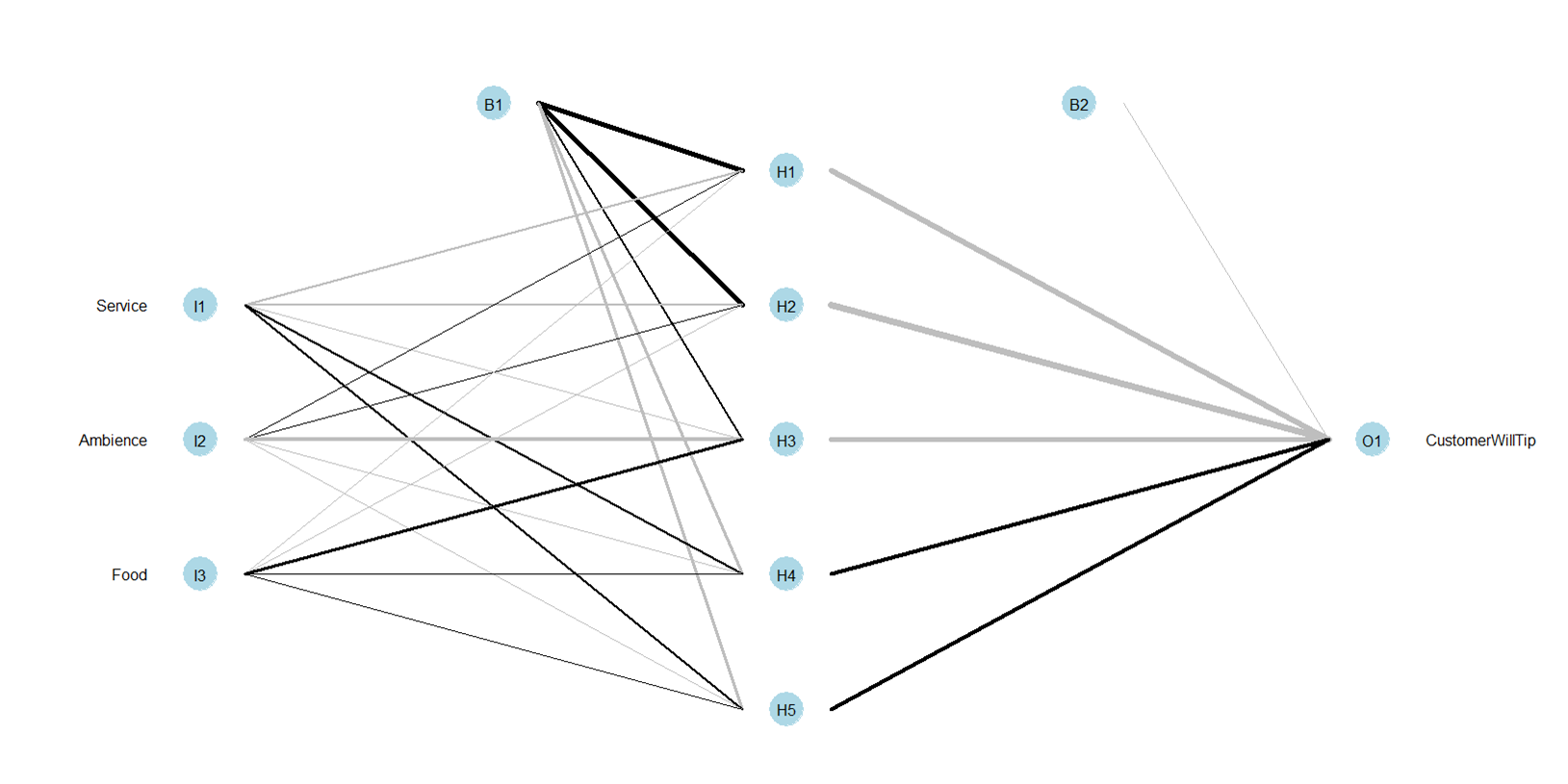Using `NeuralNetTools`, it's possible to obtain the relative importance of input variables in neural networks using `garson` algorithm:

`garson(model)`

This command prints the various input parameters and their importance to the output prediction, as shown in the following figure: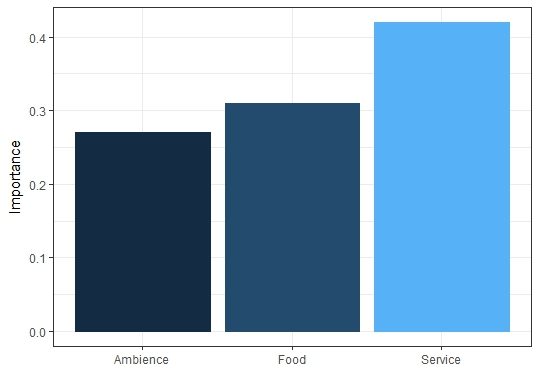From the chart obtained from the application of the Garson algorithm, it is possible to note that, in the decision to give the tip, the service received by the customers has the greater influence.

We have seen two neural network libraries in R and used them in simple examples. We would deep dive with several practical use cases throughout this book.

## Deep learning

DL forms an advanced neural network with numerous hidden layers. DL is a vast subject and is an important concept for building AI. It is used in various applications, such as:

• Image recognition
• Computer vision
• Handwriting detection
• Text classification
• Multiclass classification
• Regression problems, and more

We would see more about DL with R in the future chapters.

## Pros and cons of neural networks

Neural networks form the basis of DL, and applications are enormous for DL, ranging from voice recognition to cancer detection. The pros and cons of neural networks are described in this section. The pros outweigh the cons and give neural networks as the preferred modeling technique for data science, machine learning, and predictions.

### Pros

The following are some of the advantages of neural networks:

• Neural networks are flexible and can be used for both regression and classification problems. Any data which can be made numeric can be used in the model, as neural network is a mathematical model with approximation functions.
• Neural networks are good to model with nonlinear data with large number of inputs; for example, images. It is reliable in an approach of tasks involving many features. It works by splitting the problem of classification into a layered network of simpler elements.
• Once trained, the predictions are pretty fast.
• Neural networks can be trained with any number of inputs and layers.
• Neural networks work best with more data points.

### Cons

Let us take a look at some of the cons of neural networks:

• Neural networks are black boxes, meaning we cannot know how much each independent variable is influencing the dependent variables.
• It is computationally very expensive and time consuming to train with traditional CPUs.
• Neural networks depend a lot on training data. This leads to the problem of over-fitting and generalization. The mode relies more on the training data and may be tuned to the data.

## Best practices in neural network implementations

The following are some best practices that will help in the implementation of neural network:

• Neural networks are best implemented when there is good training data
• More the hidden layers in an MLP, the better the accuracy of the model for predictions
• It is best to have five nodes in the hidden layer
• ReLU and Sum of Square of Errors (SSE) are respectively best techniques for activation function and error deduction

## Quick note on GPU processing

The increase in processing capabilities has been a tremendous booster for usage of neural networks in day-to-day problems. GPU is a specialized processor designed to perform graphical operations (for example, gaming, 3D animation, and so on). They perform mathematically intensive tasks and are additional to the CPU. The CPU performs the operational tasks of the computer, while the GPU is used to perform heavy workload processing.

The neural network architecture needs heavy mathematical computational capabilities and GPU is the preferred candidate here. The vectorized dot matrix product between the weights and inputs at every neuron can be run in parallel through GPUs. The advancements in GPUs is popularizing neural networks. The applications of DL in image processing, computer vision, bioinformatics, and weather modeling are benefiting through GPUs.

## Summary

In this chapter, we saw an overview of ANNs. Neural networks implementation is simple, but the internals are pretty complex. We can summarize neural network as a universal mathematical function approximation. Any set of inputs which produce outputs can be made a black box mathematical function through a neural network, and the applications are enormous in the recent years.

We saw the following in this chapter:

• Neural network is a machine learning technique and is data-driven
• AI, machine learning, and neural networks are different paradigms of making machines work like humans
• Neural networks can be used for both supervised and unsupervised machine learning
• Weights, biases, and activation functions are important concepts in neural networks
• Neural networks are nonlinear and non-parametric
• Neural networks are very fast in prediction and are most accurate in comparison with other machine learning models
• There are input, hidden, and output layers in any neural network architecture
• Neural networks are based on building MLP, and we understood the basis for neural networks: weights, bias, activation functions, feed-forward, and backpropagation processing
• Forward and backpropagation are techniques to derive a neural network model

Neural networks can be implemented through many programming languages, namely Python, R, MATLAB, C, and Java, among others. The focus of this book will be building applications using R. DNN and AI systems are evolving on the basis of neural networks. In the forthcoming chapter, we will drill through different types of neural networks and their various applications.

• Giuseppe Ciaburro

Giuseppe Ciaburro holds a PhD in environmental technical physics, along with two master's degrees. His research was focused on machine learning applications in the study of urban sound environments. He works at the Built Environment Control Laboratory at the Università degli Studi della Campania Luigi Vanvitelli, Italy. He has over 15 years' professional experience in programming (Python, R, and MATLAB), first in the field of combustion, and then in acoustics and noise control. He has several publications to his credit.

Browse publications by this author
• Balaji Venkateswaran

Balaji Venkateswaran is an AI expert, data scientist, machine learning practitioner, and database architect. He has 17+ years of experience in investment banking payment processing, telecom billing, and project management. He has worked for major companies such as ADP, Goldman Sachs, MasterCard, and Wipro. Balaji is a trainer in data science, Hadoop, and Tableau. He holds a postgraduate degree PG in business analytics from Great Lakes Institute of Management, Chennai.

Balaji has expertise relating to statistics, classification, regression, pattern recognition, time series forecasting, and unstructured data analysis using text mining procedures. His main interests are neural networks and deep learning.

Balaji holds various certifications in IBM SPSS, IBM Watson, IBM big data architect, cloud architect, CEH, Splunk, Salesforce, Agile CSM, and AWS.

If you have any questions, don't hesitate to message him on LinkedIn (balvenkateswaran); he will be more than glad to help fellow data scientists.

Browse publications by this author
Latest Reviews (7 reviews total)
Pienso que es una buena introducción al tema desde el punto de vista de R.
Well written and effective
Nice introduction requiring minimal prequiste knowledge.